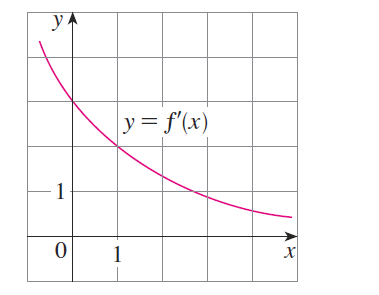#### Problem 41E

41. Suppose that the only information we have about a function $f$ is that $f(1)=5$ and the graph of its derivative is as shown.

(a) Use a linear approximation to estimate $f(0.9)$ and $f(1.1)$

(b) Are your estimates in part (a) too large or too small? Explain.